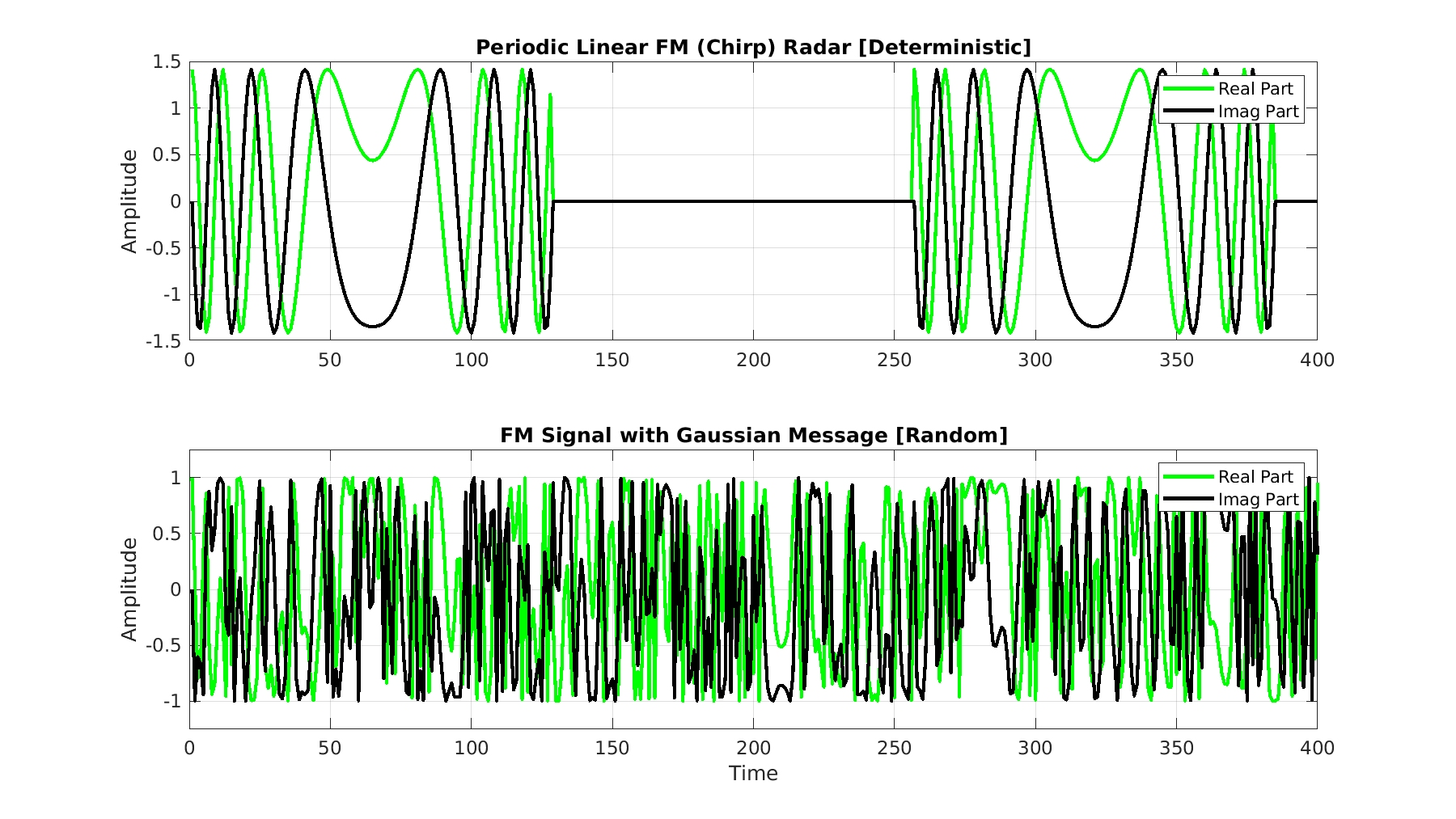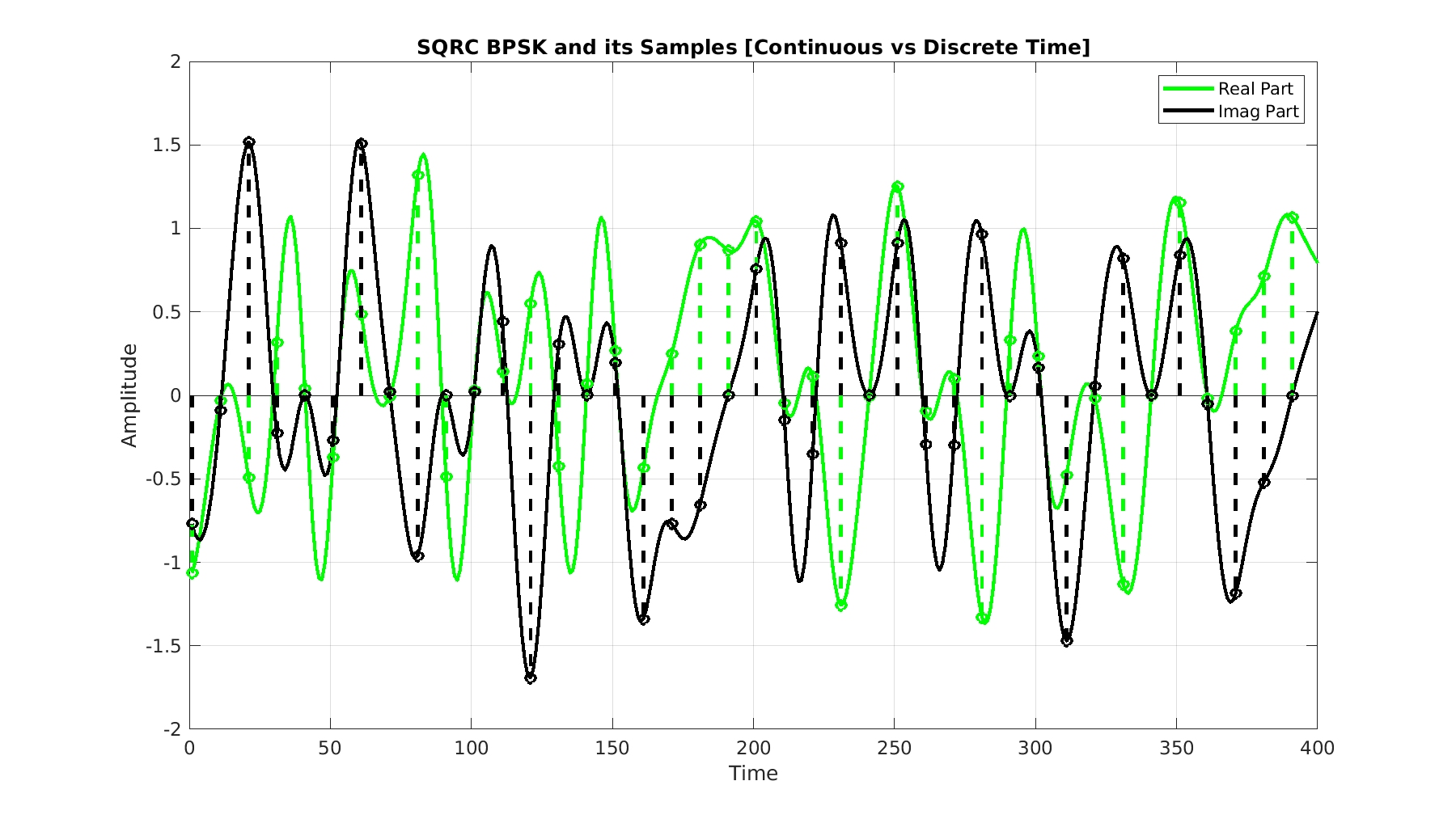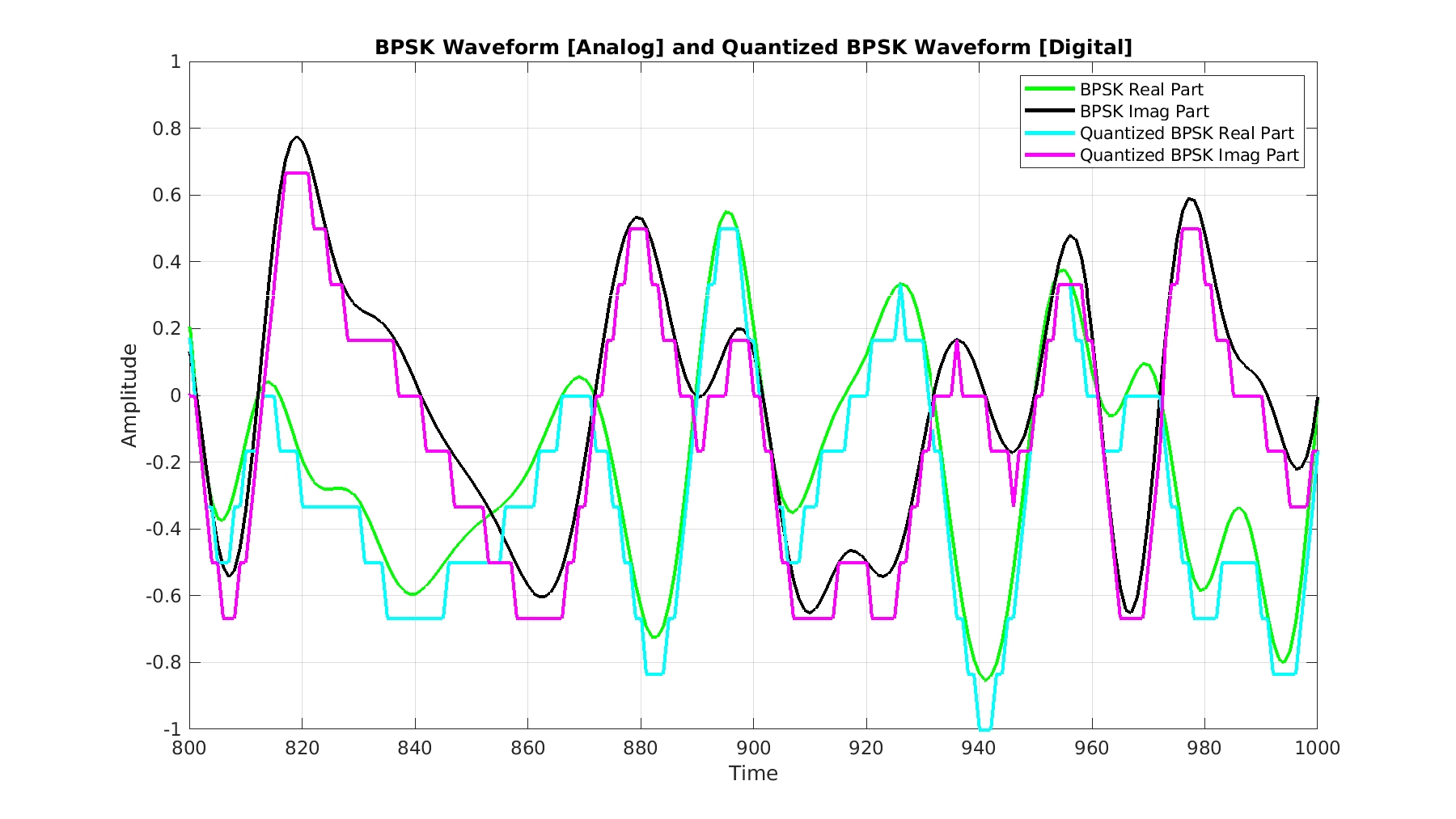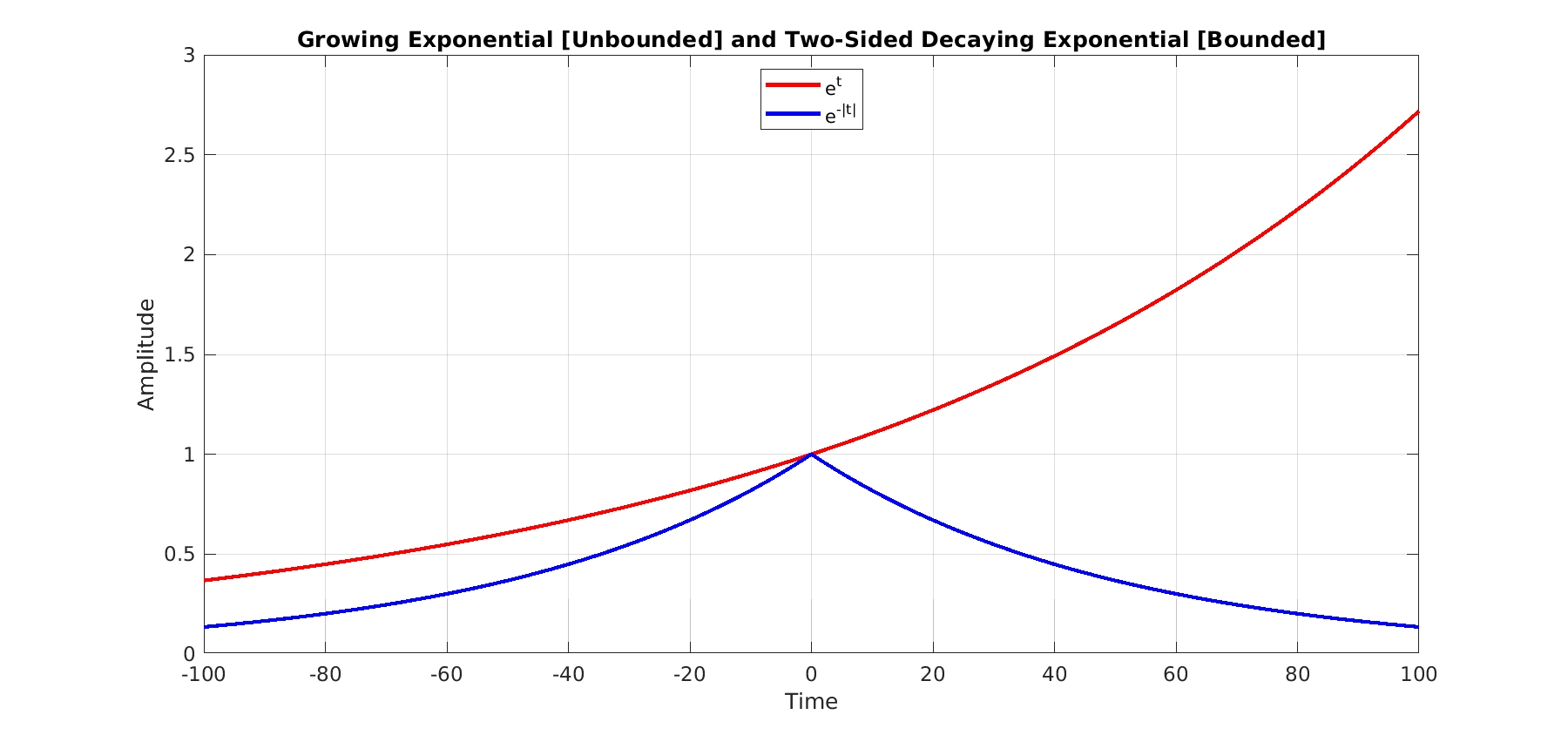# Signal Processing Toolkit: Signals

Introducing the SPTK on the CSP Blog. Basic signal-processing tools with discussions of their connections to and uses in CSP.

Next SPTK Post: Signal Representations

This is the inaugural post of a new series of posts I’m calling the Signal Processing Toolkit (SPTK).  The SPTK posts will cover relatively simple topics in signal processing that are useful in the practice of cyclostationary signal processing. So, they are not CSP posts, but CSP practitioners need to know this material to be successful in CSP. The CSP Blog is branching out! (But don’t worry, there are more CSP posts coming too.)

[Jump straight to the ‘Significance of Signals in CSP’ below.]

In this first SPTK post, we’ll discuss some basic properties of signals. In the previous CSP Blog posts, we’ve been so focused on the CSP that we didn’t bother discussing or defining or studying signals themselves. So, what do we mean by ‘signal?’

In my mind, and in my work, a signal is pretty much a function. That is, it is a quantity that varies with one or more other quantities. If we take the classic function$y = f(x)$, then$y$ is a signal, and it varies with some other quantity$x$ according to the rule defined by$f(\cdot)$. Of more general interest to us on the CSP Blog is some quantity that varies with time$t$, such as$y = s(t)$. We usually drop the$y=$ and just focus on the rule$s(t)$. The quantity$s$ can be a voltage, a current, the value of an electric field, the value of a magnetic field, etc. We are most often concerned with functions of time because a large part of our signal processing and electrical engineering work involves communicating or sensing some information across time and space using time-varying electromagnetic waves.

According to Wikipedia, “In signal processing, a signal is a function that conveys information about a phenomenon.” A lot of what comes next in this post is also found on that Wikipedia page, and elsewhere of course, such as in many books on signal processing (my favorite is The Literature [R132]).

### Kinds of Signals

It is useful to describe various signal-related dichotomies, because in our ongoing work we encounter various signals and we want to quickly assess which of our many signal-processing tools will be most appropriate and useful for application to the signal–which tools can extract the most useful information from the signal.

#### Periodic versus Aperiodic

A signal$s(t)$ is periodic if it repeats over all time$t$$\displaystyle s(t) = s(t + T_0), \ \ \ \ \forall t \hfill (1)$

where$T_0 > 0$ is called the period of the signal. If no such period$T_0$ exists, then the signal is aperiodic. Sine waves, constants, the radiation from a pulsar, and many pulse trains are periodic. Receiver noise, the bits in a communication signal, a whale song, the daily stock market value, and most other signals you can think of are aperiodic.

Aperiodic signals may contain a periodic component, meaning the signal can be accurately modeled as the sum of an aperiodic component and a periodic component, where the temporal support for the two components is disjoint. for example, a communication signal that periodically transmits the same short sequence is an aperiodic signal overall, but possesses a periodic component.

The sum of$N$ periodic signals can be periodic. The condition is that the various involved periods are commensurate. That is, each pair of periods possesses a quotient that is rational. This implies that the sum signal has a period that is a (generally distinct) multiple of each of the individual periods. If you add a sine wave with period$10$ one with period$11$, you’ll get a periodic function with period$110$.

If the periods are incommensurate, then the resulting sum signal is not periodic, but it is in some sense close to being a periodic function, and so it is called almost periodic. This comes up in CSP theory–see the work of Napolitano for the clearest explanations and historical notes I know of. I haven’t emphasized it on the CSP Blog because it doesn’t come up in practice much, at least in my practice. … Maybe I need to practice more …Figure 1. Illustration of periodic and aperiodic signals relevant to CSP. The top graph shows the real and imaginary components of a complex-valued sine wave . The middle graph shows two real-valued sine waves with irrational frequencies and their sum. The individual sine waves are periodic, but their sum is not. The bottom graph shows an aperiodic communication signal. It is not periodic because the values of the bits () that multiply the rectangular pulses are random.

#### Random versus Deterministic

A deterministic signal is one that is perfectly predictable in principle. A simple example is a signal with a known exact mathematical description, such as a sine wave or a square wave. Periodic signals are deterministic since if we know the signal over one period, we know the signal for all values of time$t$. But even seemingly random signals, such as chaotic signals, can be deterministic.

Which brings us to random signals. They are not deterministic, so they cannot be completely predicted even when you know the signal values over an infinite interval, such as$t \in (-\infty, t_0)$. There are many kinds of random signals, and they are traditionally described mathematically using random processes (also called stochastic processes). In most people’s minds, an observed (real-world) cyclostationary signal arises from a theoretical (fictitious) cyclostationary stochastic process; signal here indicates a single function of time, process indicates an infinite collection of such functions.Figure 2. Illustration of random and deterministic (non-random) signals. The top graph shows a portion of a chirp pulse train (a radar signal) that is periodic and therefore perfectly predictable. The bottom graph shows a portion of a frequency-modulated (FM) signal that is a combination of a deterministic sine-wave carrier and a random message signal, and so is random.

#### CONTINUOUS-TIME VERSUS DISCRETE-TIME

The communication signals that are at the center of the CSP Blog posts propagate through the atmosphere, through space, through walls, etc., at speeds near the speed of light in a vacuum and are accurately modeled as functions of a continuous time variable$t$–that’s the physical world.  This means that$t$ is a real number. Mathematical models for those signals are themselves functions of a real time variable$t$. However, once we sense the signal through the combination of a physical antenna, radio receiver, and sampler, we end up with a finite set of numbers. These numbers are the values of the continuous-time function (signal) at (usually) regularly spaced values of time, rather than at all values of time. That is, the continuous-time signal in the air,$s_c(t)$, becomes the discrete-time signal in a computer,$s_c(nT_s)$, where$T_s = 1/f_s$ is the sampling increment,$f_s$ is the sampling frequency, and$n$ is an integer.Figure 3. Illustration of continuous-time and discrete-time signals. Continuous-time signals are defined for all instants of time in some interval, usually . Discrete-time signals are only defined at a countable number of time instants, usually .

#### Energy versus Power

The next dichotomy relates to the integrability (continuous time) or summability (discrete time) of a signal. The difference between energy and power signals is important to understand in the context of CSP–only power signals can be cyclostationary signals.

The energy of a signal over a finite interval with length$T$ is$\displaystyle E_s(T) = \int_{t_0}^{t_0 + T} \left| s(t) \right|^2 \, dt. \hfill (2)$

Using$t_0 = -T/2$ we obtain a more typical definition$\displaystyle E_s(T) = \int_{-T/2}^{T/2} \left| s(t) \right|^2 \, dt. \hfill (3)$

Now consider calculating the energy over all time by letting$T$ grow without bound,$\displaystyle E_s = \lim_{T\rightarrow\infty} E_s(T) = \int_{-\infty}^\infty \left| s(t) \right|^2 \, dt \hfill (4)$

If this limit exists, it is called the energy of the signal$s(t)$, and the signal is an energy signal. If it doesn’t exist (for example,$E_s(T)$ increases with$T$, so that the energy grows without bound), then the signal is not an energy signal.

Any signal that has finite temporal support and is square-integrable over that support is an energy signal. Examples are a rectangular pulse, a decaying exponential$e^{-t^2}$, and a chirp pulse.

The power of a signal over an interval of length$T$ is the average energy over that interval,$\displaystyle P_s(T) = \frac{E_s(T)}{T} = \frac{1}{T} \int_{t_0}^{t_0 + T} \left| s(t) \right|^2 \, dt. \hfill (4)$

If the power measured over all time exists and is not zero,$\displaystyle P_s = \lim_{T\rightarrow\infty} P_s(T) > 0 \hfill (5)$

then the signal is a power signal.

Roughly speaking, energy signals are transient and power signals are persistent (another dichotomy). Examples of power signals are the rectangular-pulse BPSK signal, the various square-root raised-cosine digital QAM/PSK signals, Gaussian noise, etc.Figure 4. Illustration of power and energy signals. Power signals have infinite energy and persist to infinity in the time dimension. Energy signals have zero power and typically have finite support in time. But this is not required-some energy signals are non-zero for all time but still have zero power, such as the Gaussian signal in the figure.

#### Stationary versus Nonstationary

The concept of stationarity applies, formally, to random processes. The basic idea is that a stationary signal possesses probabilistic parameters that are time-invariant. A probabilistic parameter is something like a mean value, the autocorrelation,$n$th-order moments,$n$th-order joint probability distribution and density functions, etc. For a stationary signal, such parameters do not depend on the time variable$t$. If we look at the mean value of$s(t_1)$ (over all the sample paths making up the random process), it will be the same as that for$s(t_2)$, for all possible$t_1$ and$t_2$. An interpretation is that the signal ‘looks the same,’ in a probabilistic sense, no matter where in time you choose to look at it.

For nonstationary signals, at least some of the probabilistic parameters are not time invariant. For cyclostationary signals, at least some probabilistic parameters are periodically or almost periodically time variant, which is a special case of nonstationarity.

#### ANALOG VERSUS DIGITAL

This dichotomy concerns the values that the signal can take on rather than the values that the independent variable (typically time$t$) can take on. For analog signals, the possible values of the signal are real numbers in some interval (for real-valued signals) or complex numbers in some closed area (for complex-valued signals).

Digital signals, on the other hand, can only take on values in some finite set. An example of this is the output of an analog-to-digital converter. The continuous-time analog signal enters the device and what comes out is a discrete-time signal that can take on values that are constrained to lie in a set that is determined by the number of bits used to represent and approximate the obtained sample values.Figure 5. Illustration of analog and digital signals. Analog signals can take on one of an uncountable infinity of values at each time instant. For example, they might take on any value in or in . Digital signals can only take on a one of a finite number of values in some set, which is often called the signal’s alphabet. Analog and digital signals can be continous-time or discrete-time signals.

#### Of Interest versus Not of Interest

In communications-signal processing, we often encounter situations that are characterized by multiple signals that overlap in both time and frequency. Typically one is ‘our’ signal, and the others are interfering signals. You’ll see people refer to ‘our’ signal as the ‘signal of interest’ (SOI) and the other signals as ‘signals not of interest’ (SNOIs). Sometimes we want to know the statistical structure of all the signals to facilitate processing that attempts to extract the SOI (FRESH filtering), and sometimes we don’t need to know much about the SNOI to deal with it (notch filtering).Figure 6. Illustration of signals of interest and of no interest (or ‘not of interest’). Signals of interest, SOIs, are defined as those signals at which our processing is aimed, irrespective of the transmitter intent. Signals not of interest are all other signals. A typical situation involves a communication receiver. The transmitter sends a signal that is intended for the receiver, which is the signal of interest, but other signals may occupy the band, which are interferers.

#### Bounded versus Unbounded

A bounded signal$x(t)$ is one that satisfies the following condition$\displaystyle \left| x(t) \right| < M \ \ \ \forall t, \hfill (6)$

where$M$ is a finite positive number. Bounded signals include all sinusoids, rectangular pulse trains, two-sided decaying exponentials such as$e^{-|t|}$, etc. Unbounded signals include the impulse function and increasing exponentials such as$e^t$.Figure 7. Illustration of bounded and unbounded signals. Bounded signals are those whose magnitude never exceeds some finite (but maybe large) number . If a signal is not bounded, it is unbounded. Unbounded signals typically occur when feedback goes awry, causing the output of some circuit to increase without cease.

If you think of a signal dichotomy that is important and that I’ve not covered here, please leave a note in the Comments.

### Significance of Signals in CSP

Cyclostationary signals are power signals. Periodic signals are power signals that are trivially cyclostationary (why trivial?). Much of our theoretical work in CSP focuses on analog signals, while our practical CSP work focuses almost exclusively on discrete-time digital signals. Energy signals have no theoretical cyclostationarity–everything is averaged away. But since any finite segment of a power signal is an energy signal, and we can reliably measure the cyclic features of long-enough energy signals, energy signals are important in practice.